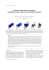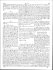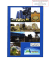# How to Find Discrete Contact Symmetries

## Transcription

How to Find Discrete Contact Symmetries
```Journal of Nonlinear Mathematical Physics
1998, V.5, N 4, 405–416.
Article
How to Find Discrete Contact Symmetries
Peter E. HYDON
Department of Mathematics and Statistics,
University of Surrey, Guildford GU2 5XH, UK
E-mail: [email protected]
Received May 15, 1998; Accepted July 7, 1998
Abstract
This paper describes a new algorithm for determining all discrete contact symmetries
of any differential equation whose Lie contact symmetries are known. The method
is constructive and is easy to use. It is based upon the observation that the adjoint
action of any contact symmetry is an automorphism of the Lie algebra of generators
of Lie contact symmetries. Consequently, all contact symmetries satisfy various compatibility conditions. These conditions enable the discrete symmetries to be found
systematically, with little effort.
1.
Introduction
Discrete symmetries of differential equations are used in various ways. They map solutions
to (possibly new) solutions. They may be used to create efficient numerical methods for
the computation of solutions to boundary-value problems. Indeed, there is currently much
research into techniques for constructing numerical methods that preserve various types of
symmetry [2, 4, 10]. Discrete and continuous groups of symmetries determine the nature
of bifurcations in nonlinear dynamical systems. Equivariant bifurcation theory describes
the effects of symmetries, but it may yield misleading results unless all symmetries of the
dynamical system are known [3, 6].
In general, it is straightforward to find all one-parameter Lie groups of symmetries
of a given system, using techniques developed by Sophus Lie more than a century ago
[1, 11, 12, 14]. Yet, until recently, no simple method for finding all discrete symmetries
was known. Ansatz-based methods can be used to find discrete symmetries belonging to
particular classes, e.g. , but such methods cannot guarantee that all discrete symmetries
have been found. The main difficulty is that, commonly, the determining equations for
discrete symmetries form a highly-coupled nonlinear system. Reid and co-workers have
developed a computer algebra package aimed at reducing this system to a differential
Gr¨obner basis , but the method is computationally intensive and seems not to have
been widely used.
c
by P.E. Hydon
406
P.E. Hydon
A new approach to the problem of finding discrete point symmetries has recently been
described by the author . Instead of trying to solve the symmetry condition directly,
one first examines the adjoint action of an arbitrary discrete point symmetry upon the
Lie algebra of Lie point symmetry generators. This yields a set of necessary conditions
which simplify the problem of constructing all discrete point symmetries. Of course,
one must know the Lie algebra of Lie point symmetry generators, which must be nontrivial. However, this is not a severe limitation, as most differential equations of physical
importance have a non-trivial Lie algebra.
Most techniques of Lie symmetry analysis readily generalize to contact symmetries,
and the aim of the current paper is to show how all discrete contact symmetries of a given
differential equation may be found systematically, using an extension of the algorithm for
determining discrete point symmetries. Perhaps surprisingly, many differential equations
without non-point Lie contact symmetries have non-point discrete contact symmetries. It
is useful to be able to derive these (non-obvious) symmetries systematically because, like
all symmetries, they constrain the behaviour of solutions of the differential equation.
The new method is described first in the context of ordinary differential equations
(ODEs) of order n ≥ 3, then adapted to treat a finite subgroup of the infinite group of
contact symmetries of a given second-order ODE. Finally, it is shown that the method can
be extended to partial differential equations (PDEs).
2.
The algorithm applied to ODEs
A diffeomorphism
Γ : (x, y) 7→ (ˆ
x, yˆ)
is a symmetry of the ODE
´
³
y (n) = ω x, y, y ′ , . . . , y (n−1)
if it maps the set of solutions to itself, i.e. if
³
´
yˆ(n) = ω x
ˆ, yˆ, yˆ′ , . . . , yˆ(n−1)
when (2.1) holds.
(2.1)
(2.2)
Here the functions yˆ(k) are obtained by prolonging the diffeomorphism Γ to derivatives,
using
³
´
Dˆ
y (k−1)
yˆ(k) ≡
,
yˆ(0) = yˆ ,
(2.3)
Dˆ
x
where
D = ∂x + y ′ ∂y + y ′′ ∂y′ + · · · .
For point symmetries, x
ˆ and yˆ are functions of x and y. Contact symmetries are more
general than point symmetries, because x
ˆ, yˆ and yˆ′ are functions of x, y and y ′ . Oneparameter Lie groups of contact symmetries are obtained in a similar way to Lie point
symmetries, by linearizing the symmetry condition (2.2). Specifically,
¡ ¢
x
ˆ = x + ǫξ(x, y, y ′ ) + O ǫ2 ,
¡ ¢
yˆ = y + ǫη(x, y, y ′ ) + O ǫ2 ,
¡
¢
¡ ¢
k ≥ 1.
yˆ(k) = y (k) + ǫη (k) x, y, y ′ , . . . , y (k) + O ǫ2 ,
How to Find Discrete Contact Symmetries
407
The contact conditions require that the O(ǫ) terms should be expressible in terms of the
characteristic function Q(x, y, y ′ ) = η − y ′ ξ, as follows
ξ = −Qy′ ,
η = Q − y ′ Qy′ ,
η (k) = Dk Q − y (k+1) Qy′ ,
k ≥ 1.
(2.4)
In particular,
η (1) (x, y, y ′ ) = Qx + y ′ Qy .
Lie point symmetries of ODEs of order n ≥ 2 are Lie contact symmetries whose characteristic function is linear in y ′ .
The set of all infinitesimal generators of Lie contact symmetries of a given ODE of
order n ≥ 3 forms a finite-dimensional Lie algebra, L, which can generally be determined
systematically . Given such an ODE, suppose that L has a basis
(1)
Xi = ξi (x, y, y ′ )∂x + ηi (x, y, y ′ )∂y + ηi (x, y, y ′ )∂y′ ,
i = 1, . . . , N,
(2.5)
´
³
(1)
are obtained from the characteristic function Qi (x, y, y ′ ) using (2.4),
where ξi , ηi , ηi
and N = dim (L). The structure constants, ckij , for the basis (2.5) are determined by
[Xi , Xj ] = ckij Xk .
(2.6)
(Summation is implied when an index occurs twice, one raised and once lowered.) The oneparameter Lie group of contact symmetries corresponding to a particular Xi is obtained
by exponentiation. We use the notation
¢
¡
Γi (ǫ) : (x, y, y ′ ) 7→ eǫXi x, eǫXi y, eǫXi y ′ .
Suppose that
¡
¢
Γ : (x, y, y ′ ) 7→ x
ˆ(x, y, y ′ ), yˆ(x, y, y ′ ), yˆ′ (x, y, y ′ )
(2.7)
ˆ i (ǫ) = ΓΓi (ǫ)Γ−1 ,
Γ
(2.8)
is a contact symmetry of the given ODE. Then the contact transformation obtained by
the adjoint action of Γ upon Γi (ǫ),
is also a contact symmetry, for each ǫ in some neighbourhood of zero. Therefore, for each i,
there is a (local) one-parameter Lie group of contact symmetries
´
³
ˆ
ˆ
ˆ
ˆ i (ǫ) : (ˆ
(2.9)
Γ
x, yˆ, yˆ′ ) 7→ eǫXi x
ˆ, eǫXi yˆ, eǫXi yˆ′ ,
whose infinitesimal generator is
(1)
ˆ i = ΓXi Γ−1 = ξi (ˆ
X
x, yˆ, yˆ′ )∂xˆ + ηi (ˆ
x, yˆ, yˆ′ )∂yˆ + ηi (ˆ
x, yˆ, yˆ′ )∂yˆ′ .
(2.10)
Consequently
ˆ i ∈ L,
X
i = 1, . . . , N.
ˆ i }N are simply the basis generators {Xi }N with (x, y, y ′ ) replaced
The generators {X
i=1
i=1
ˆ i }N is a basis for L, and so each Xi can be written as
by (ˆ
x, yˆ, yˆ′ ). Therefore the set {X
i=1
408
P.E. Hydon
ˆ j ’s. Also, the mapping Xi 7→ X
ˆ i is an automorphism of L
a linear combination of the X
which preserves all structure constants, i.e.
ˆk
ˆi, X
ˆ j ] = ckij X
[X
when (2.6) holds.
(2.11)
These results generalize to partial differential equations, and are summarized as follows.
Lemma 1. Every contact symmetry Γ of an ordinary differential equation of order n ≥ 3
induces an automorphism of the Lie algebra, L, of generators of one-parameter local Lie
groups of contact symmetries ¡of ¢the differential equation. For each such Γ, there exists a
constant non-singular matrix bli such that
ˆl .
Xi = bli X
(2.12)
This automorphism preserves all structure constants.
Lemma 1 yields the following PDEs for the unknown functions x
ˆ(x, y, y ′ ) and yˆ(x, y, y ′ ):
ˆl x
x, yˆ, yˆ′ ),
ˆ = bli ξl (ˆ
Xi x
ˆ = bli X
i = 1, . . . , N,
(2.13)
ˆ l yˆ = bli ηl (ˆ
Xi yˆ = bli X
x, yˆ, yˆ′ ),
i = 1, . . . , N.
(2.14)
This set of 2N first-order PDEs, together with the contact condition
dˆ
y
yˆ′ (x, y, y ′ ) = dx ,
dˆ
x
dx
provides necessary, but not sufficient, conditions for Γ to be a contact symmetry. The
contact condition yields the following pair of PDEs, because yˆ′ is independent of y ′′ :
yˆx + y ′ yˆy = (ˆ
xx + y ′ x
ˆy )ˆ
y′ ,
ˆy′ yˆ′ .
yˆy′ = x
(2.15)
N.B. The lemma gives a further N PDEs
ˆ l yˆ′ = bli η (1) (ˆ
Xi yˆ′ = bli X
x, yˆ, yˆ′ ),
l
i = 1, . . . , N,
but these add nothing new, for they are a consequence of (2.13), (2.14) and the contact
condition.
To find all discrete contact symmetries, proceed as follows. First solve the system of
PDEs (2.13), (2.14), to obtain (ˆ
x, yˆ) in terms of x, y, y ′ , bli and some unknown constants
(or functions) of integration. If N is sufficiently large, it may be possible to solve this
system algebraically (by eliminating the derivative terms); otherwise, the method of characteristics should be used. Incorporate the contact condition (2.15); this generally reduces
the number of candidate solutions (ˆ
x, yˆ). Finally, use the symmetry condition (2.2) to
determine which of these solutions are symmetries. The continuous symmetries may be
factored out at a convenient point in the calculation. The remaining discrete symmetries
are inequivalent under any continuous symmetry, and form a discrete (but not necessarily
finite) group.
If ¡L ¢is non-abelian, some of the structure constants are non-zero, enabling the matrix
B = bli to be simplified before any of the above calculations are done. Substituting (2.12)
How to Find Discrete Contact Symmetries
409
into (2.6), and taking (2.11) into account, we obtain the following constraints on the
components of B:
k n
cnlm bli bm
j = cij bk .
(2.16)
It is sufficient to restrict attention to equations (2.16) with i < j, because the structure
constants are antisymmetric in the two lower indices. Moreover, at least some of the
continuous symmetries can be factored out using their adjoint action upon the generators
in L, (see ),
ǫ2j
[Xj , [Xj , Xi ]] − · · · = api (ǫj , j)Xp .
(2.17)
2!
Let A(j) denote the matrix whose components are api (ǫj , j), as defined by (2.17). The
system (2.12) is equivalent, under the group generated by Xj , to
ˆl ,
Xi = ˜bli X
Ad(exp(ǫj Xj ))Xi = Xi − ǫj [Xj , Xi ] +
where ˜bli are the components of
˜ = A(j)B.
B
(2.18)
˜ does not affect (2.16), so we will drop tildes as soon as each
The mapping B 7→ B
equivalence transformation has been made. Each generator Xj is used in turn to simplify
the form of B.
To illustrate this procedure, consider the two-dimensional non-abelian Lie algebra a(1),
with a basis {X1 , X2 } such that
[X1 , X2 ] = X1 .
(2.19)
The only non-zero structure constants are
c112 = −c121 = 1.
Therefore (2.16) gives
b11 b22 − b21 b12 = b11 ,
0 = b21 ,
and hence
b1
B = 11
b2
·
¸
0
,
1
b11 6= 0.
The matrices representing the adjoint action of the continuous group on the Lie algebra
are
·
¸
· ǫ
¸
1
0
e2 0
A(1) =
,
A(2) =
.
−ǫ1 1
0 1
Applying (2.18), first with j = 1, ǫ1 =
·
α
B=
0
¸
0
,
1
where
b12
, then with j = 2, ǫ2 = − ln |b11 |, we obtain
b11
α ∈ {−1, 1}.
(2.20)
The reduced form of the matrix B is specific to this particular Lie algebra, and is independent of the ODE whose Lie point symmetries are generated by the algebra.
Simplified matrices for other non-abelian Lie algebras can be found by the same technique. However, if L is abelian, the entries of B cannot be determined a priori.
410
P.E. Hydon
3.
Examples
The third-order ODE
y ′′′ =
y ′′2 y ′′
− ′
x
y
(3.1)
has a two-dimensional Lie algebra of generators of Lie contact symmetries. These Lie
symmetries are actually point symmetries, and L is isomorphic to a(1). The basis of L,
X1 = ∂y ,
X2 =
y′
x
∂x + y∂y + ∂y′ ,
2
2
has the commutation relations (2.19), and therefore B is given by (2.20). The system of
PDEs (2.13), (2.14) amounts to

 

·
¸ ·
¸ 0 1
0 α
X1 x
ˆ X1 yˆ
α 0 
=
.
=
x
ˆ
x
ˆ
X2 x
ˆ X2 yˆ
0 1
yˆ
yˆ
2
2
The general solution of this system is
x
ˆ = xp(t),
yˆ = αy + x2 q(t),
where
t=
y′
.
x
The contact condition gives
yˆ′ = xr(t),
where
αt + 2q − tq˙ = (p − tp)r
˙
and
q˙ = pr.
˙
(Here a dot over a function denotes its derivative with respect to t.) Re-arranging these
conditions, we obtain
q=
1
(pr − αt) ,
2
(3.2)
and the contact condition is satisfied if and only if
pr˙ − pr
˙ = α.
(3.3)
It is convenient to work in terms of p and r, because the ODE is invariant under translations
in y, and so y does not occur in the symmetry condition. The prolongation to second and
third derivatives is
yˆ′′ =
r + (y ′′ − t)r˙
,
p + (y ′′ − t)p˙
yˆ′′′ =
′′′ + (y ′′ − t)2 (p¨
(pr˙ − pr)xy
˙
r − p¨r) + (y ′′ − t)3 (p¨
˙r − p¨r)
˙
.
x(p + (y ′′ − t)p)
˙ 3
These expressions are substituted into the symmetry condition (2.2), and powers of y ′′ are
equated to yield an over-determined system of nonlinear ODEs for p and r. These are
How to Find Discrete Contact Symmetries
411
easily solved with the aid of the contact condition (3.3). There are two sets of solutions.
Either
(p, r) = (c, ct),
c2 = α,
(3.4)
(p, r) = (ct, c),
c2 = −α.
(3.5)
or
Re-writing these solutions in terms of the original variables, we obtain eight inequivalent
discrete symmetries that form a group isomorphic to Z4 × Z2 . The group generators are
Γ1 : (x, y, y ′ ) 7→ (ix, −y, iy ′ ),
Γ2 : (x, y, y ′ ) 7→ (y ′ , xy ′ − y, x).
The subgroup generated by Γ1 consists of four discrete point symmetries, which can be
found without having to consider contact symmetries . The four inequivalent non-point
contact symmetries are obtained from the point symmetries by composition with Γ2 , which
is the prolonged Legendre Transform.
Generally speaking, the larger the dimension of L, the easier it is to find the discrete
symmetries. Nevertheless, the contact condition makes it possible to solve the governing
equations, even when N = 1. Suppose that L is one-dimensional and the ODE is written
in canonical coordinates, so that the continuous symmetries are generated by
X = ∂y .
ˆ where b 6= 0, and therefore
Then Lemma 1 gives X = bX,
x
ˆ = f (x, y ′ ),
yˆ = by + g(x, y ′ ).
The contact condition yields
yˆ′ = h(x, y ′ ),
where
gx = fx h − by ′ ,
gy′ = fy′ h.
(3.6)
Equations (3.6) are compatible if and only if
fx hy′ − fy′ hx = b.
(3.7)
They can be integrated, once f and h are known, to determine g up to an arbitrary
constant, which may be set at any convenient value to factor out equivalence under the
one-parameter group generated by X.
Consider the general third order ODE admitting the group generated by X:
y ′′′ = ω(x, y ′ , y ′′ ).
Substituting
x
ˆ = f (x, y ′ ),
yˆ′ = h(x, y ′ )
412
P.E. Hydon
into the symmetry condition, and then equating powers of y ′′ , yields an over-determined
coupled system of nonlinear PDEs. This system is precisely as intractible as the problem
of using the symmetry condition alone to find all point symmetries of
y ′′ = ω(x, y, y ′ ).
However, with the aid of the contact condition (3.7), the problem simplifies considerably.
To illustrate this, consider the ODE
′′′
y =y
′′3
sin
µ
x
y′
¶
(3.8)
,
whose only Lie contact symmetries are those generated by X = ∂y . The symmetry condition gives the over-determined (but complicated) system
fx hxx − fxx hx =
h3x sin
µ ¶
f
,
h
fy′ hxx + 2fx hxy′ − fxx hy′ − 2fxy′ hx =
fx h
y′ y′
+ 2f h
y′
xy ′
−f
y′ y′
hx − 2f
xy ′
3h2x hy′
h =
y′
fy′ hy′ y′ − fy′ y′ hy′ + (fx hy′ − fy′ hx ) sin
µ
µ ¶
f
sin
,
h
µ ¶
f
,
sin
h
3hx h2y′
x
y′
¶
=
h3y′
µ ¶
f
sin
.
h
This system can be greatly simplified by using (3.7) and its differential consequences,
which reduces the first three equations to
fxx = fxy′ = hx = hy′ y′ = 0.
Combining this result with (3.7) and the remaining symmetry condition gives
f = α(x + 2nπy ′ ),
h = y′,
α ∈ {−1, 1},
n ∈ Z.
After solving (3.6) for g(x, y ′ ) and setting g(0, 0) = 0 to factor out the continuous symmetries, we obtain the following result. The inequivalent discrete contact symmetries of
(3.8) form a countably-infinite group, which is generated by
Γ1 : (x, y, y ′ ) 7→ (−x, −y, y ′ ),
Γ2 : (x, y, y ′ ) 7→ (x + 2πy ′ , y + πy ′2 , y ′ ).
How to Find Discrete Contact Symmetries
4.
413
Discrete uniform contact symmetries
For ODEs of order n ≥ 3, the Lie contact symmetries can generally be found systematically,
and L is finite-dimensional. Second-order ODEs have an infinite-dimensional Lie algebra
of contact symmetry generators, but they cannot all be found unless the general solution
of the ODE is known. However, some contact symmetries may be found with the aid
of a suitable ansatz for Q. For example, the restriction Qy′ y′ = 0 enables all Lie point
symmetries to be found systematically.
Other restrictions on Q are possible. For example, the set of all uniform contact
symmetries of a given ODE of order n ≥ 2 is a finite-dimensional Lie group . Uniform
contact symmetries are of the form
x
ˆ = Φ(x, y ′ ),
yˆ = ky + Θ(x, y ′ ),
yˆ′ = Ψ(x, y ′ ),
k ∈ R\{0},
(4.1)
where the contact condition requires that
ky ′ + Θx = Φx Ψ,
Θy′ = Φy′ Ψ.
(4.2)
Many of the most commonly-occurring contact symmetries are uniform, including all contact symmetries of the ODEs that were used as examples in the previous section. The
generators of uniform Lie contact symmetries have characteristic functions of the form
Q = c1 y + φ(x, y ′ ),
c1 ∈ R.
The Lie algebra of these generators can be found systematically, for a given ODE of order
n ≥ 2, by equating powers of y in the symmetry condition. The constructions leading to
Lemma 1 can be repeated, restricting attention to uniform contact symmetries, to obtain
the following.
Lemma 2. Every uniform contact symmetry Γ of an ordinary differential equation of
order n ≥ 2 induces an automorphism of the Lie algebra, L, of generators of one-parameter
local Lie groups of uniform contact symmetries¡of¢the differential equation. For each such
Γ, there exists a constant non-singular matrix bli such that
ˆl .
Xi = bli X
(4.3)
This automorphism preserves all structure constants.
This lemma can be used to find all discrete uniform contact symmetries in exactly the
same way as Lemma 1 is used. To illustrate this, consider the ODE
y ′′ =
y ′2
,
3xy ′ − 4y
(4.4)
which has a four-dimensional Lie algebra of uniform contact symmetry generators. The
structure constants are simplest in the basis
X1 = 2y ′ ∂x + y ′2 ∂y ,
3
1
1
X2 = x∂x + y∂y − y ′ ∂y′ ,
4
2
4
X3 = 2(y ′ )−3 ∂x + 3(y ′ )−2 ∂y ,
414
P.E. Hydon
1
1
1
X4 = x∂x + y∂y + y ′ ∂y′ .
4
2
4
The only non-zero structure constants are
c112 = −c121 = 1,
c334 = −c343 = 1,
and so the Lie algebra is isomorphic to a(1) ⊕ a(1). After simplifying B as far as possible,
using the relations (2.16) and the adjoint action of the continuous group, we obtain two
possibilities. Either

0 0
1 0
0 β
0 0

0
0
,
0
1
α, β ∈ {−1, 1},
(4.5)

0
0
0
1

0
1
,
0
0
α, β ∈ {−1, 1}.
(4.6)
α
0
B=
0
0
or
0
0
B=
β
0
α
0
0
0
The inequivalent discrete uniform contact symmetries are calculated in the same way as
previously. Lemma 2 is used, together with (4.1) and the contact condition (4.2), to obtain
the most general form possible for a uniform contact symmetry of (4.4). The symmetry
condition for the ODE is then used to determine which of the possible solutions actually are
symmetries. We find that the group of inequivalent discrete uniform symmetries of (4.4)
is isomorphic to Z2 × Z2 × Z2 , and is generated by
Γ1 : (x, y, y ′ ) 7→ (−x, y, −y ′ ),
Γ2 : (x, y, y ′ ) 7→ (x, −y, −y ′ ),
¡
¢
Γ3 : (x, y, y ′ ) 7→ xy ′2 , 2xy ′ − y, (y ′ )−1 .
The discrete point symmetries generated by Γ1 and Γ2 can also be found directly from
the Lie algebra of point symmetries, which is Span(X2 , X4 ). These inequivalent discrete
symmetries are derived from the matrix B in (4.5), with α = β; they map each of the a(1)
Lie subalgebras to itself. However Γ3 , which is derived from (4.6), interchanges these two
subalgebras. Actually, (4.4) is merely one representative of a whole class of second-order
ODEs that have inequivalent uniform discrete symmetries Γi , i = 1, 2, 3; these ODEs are
of the form
y ′′ =
my ′2
,
y − (m + 1)xy ′
m ∈ R\{0}.
How to Find Discrete Contact Symmetries
5.
415
Contact symmetries of a nonlinear PDE
The method described in section 2 generalizes to PDEs without difficulty; the corresponding algorithm for point symmetries will be discussed elsewhere . The basic steps are
shown here, using the potential hyperbolic heat equation
uxx
(5.1)
utt + ut =
ux
as an example. The Lie algebra of contact symmetry generators is five-dimensional, with
a basis
X1 = ∂t ,
X2 = ∂ x ,
X3 = ∂ u ,
X4 = e−t ∂u − e−t ∂ut ,
X5 = −x∂x + u∂u + ut ∂ut + 2ux ∂ux .
These Lie contact symmetries are actually point symmetries, but there is still the possibility that some discrete contact symmetries may be non-point symmetries, cf. (3.1).
Using (2.16) and the adjoint action of the continuous group to simplify B, we obtain two
possibilities. Either
B = diag {1, b, α, β, 1},
α, β ∈ {−1, 1},
b ∈ R\{0},
(5.2)
or

1 0
0 0

B=
0 α
0 0
2 0
0 0
b 0
0 0
0 β
0 0

0
0 

0 
,
0 
−1
α, β ∈ {−1, 1},
b ∈ R\{0}.
(5.3)
If B is of the form (5.2), the equations analogous to (2.13), (2.14) are




1 0
0
ˆ X1 u
ˆ
X1 tˆ X1 x
0 1
 X2 tˆ X2 x
0 
ˆ X2 u
ˆ






 X3 tˆ X3 x
1 
ˆ X3 u
ˆ = B 0 0
,

 0 0 e−tˆ 
 X4 tˆ X4 x
ˆ X4 u
ˆ
0 −ˆ
x u
ˆ
X5 tˆ X5 x
ˆ X5 u
ˆ
whose general solution is
tˆ = t + c1 ,
1
x
ˆ = bx + c2 |ux |− 2 ,
1
u
ˆ = αu + (α − βe−c1 )ut + c3 |ux | 2 ,
ci ∈ R.
The contact condition and the symmetry condition reduce these further, to
(tˆ, x
ˆ, u
ˆ) = (t, αx, αu),
α ∈ {−1, 1}.
The remaining inequivalent discrete contact symmetries are obtained from (5.3) in a similar
way. To summarize the results: the inequivalent discrete contact symmetries of (5.1) form
a group isomorphic to Z2 × Z2 , which is generated by
Γ1 : (t, x, u, ut , ux ) 7→ (t, −x, −u, −ut , ux ),
¢
¡
Γ2 : (t, x, u, ut , ux ) 7→ t + ln |ux |, u + ut , x + ut (ux )−1 , −ut (ux )−1 , (ux )−1 .
The symmetry Γ2 was known previously , but it was not known that Γ2 and Γ1 Γ2 are
the only non-point contact symmetries (up to equivalence). The method outlined in the
current paper enables the user to completely classify all contact symmetries of a given
differential equation with a known non-trivial Lie algebra.
416
P.E. Hydon
References
 Bluman G.W. and Kumei S., Symmetries and Differential Equations, Springer, New York, 1989.
 Budd C.J. and Collins G.J., An Invariant Moving Mesh Scheme for the Nonlinear Diffusion Equation,
Appl. Num. Math., 1998, V.26, 23–39.
 Crawford J.D., Golubitsky M., Gomes M.G.M., Knobloch E. and Stewart I.N., Boundary Conditions as Symmetry Constraints, in Singularity Theory and its Applications, Editors M. Roberts and
I. Stewart, Warwick 1989, part II, Springer, Berlin, 1991, 63–79.
 Dorodnitsyn V., Finite Difference Methods Entirely Inheriting the Symmetry of the Original Equations, in Modern Group Analysis: Advanced Analytical and Computational Methods in Mathematical
Physics, Editors N.H. Ibragimov, M. Torrisi and A. Valenti, Kluwer, Dordrecht, 1993, 191–201.
 Gaeta G. and Rodr´ıguez M.A., Determining Discrete Symmetries of Differential Equations, Nuovo
Cimento, 1996, V.111B, 879–891.
 Golubitsky M., Stewart I. and Schaeffer D.G., Singularities and Groups in Bifurcation Theory, Vol.II,
Springer, New York, 1988.
 Hydon P.E., Discrete Point Symmetries of Ordinary Differential Equations, Proc. Roy. Soc. Lond.
A, 1998 (in press).
 Hydon P.E., Uniform Contact Symmetries of Ordinary Differential Equations (in preparation).
 Hydon P.E., Discrete Symmetries and Equivalence Transformations of Partial Differential Equations
(in preparation).
 McLachlan R.I., Quispel G.R.W. and Turner G.S., Numerical Integrators that Preserve Symmetries
and Reversing Symmetries, SIAM J. Numer. Anal., 1998, V.35, 586–599.
 Olver P.J., Applications of Lie Groups to Differential Equations, Springer, New York, 1986.
 Ovsiannikov L.V., Group Analysis of Differential Equations, Academic, New York, 1982.
 Reid G.J., Weih D.T. and Wittkopf A.D., A Point Symmetry Group of a Differential Equation which
Cannot be Found Using Infinitesimal Methods, in Modern Group Analysis: Advanced Analytical and
Computational Methods in Mathematical Physics, Editors N.H. Ibragimov, M. Torrisi and A. Valenti,
Kluwer, Dordrecht, 1993, 311–316.
 Stephani H., Differential Equations: Their Solution Using Symmetries, Cambridge University Press,
Cambridge, 1989.
 Svirshchevskii S.R., Evolution Equations I: Diffusion Equations, in CRC Handbook of Lie Group
Analysis of Differential Equations, Editor N.H. Ibragimov, Vol.1, CRC Press, Boca Raton, 1994,
102–176.
```

### The Icosahedron - Singapore Mathematical Society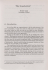### Symmetry for Architectural Design - Computer Graphics at Stanford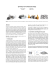### Weaving Symmetry of the Philippine Northern Kankana-ey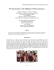### Scary Kids Scaring Kids My Knife, Your Throat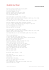### Non-Verbal Communications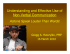### Scripture Memorization - Saxe Gotha Presbyterian Church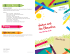### Aljunied-Hougang-Punggol East Town Council`s Audited Financial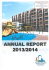### Probably Approximately Symmetric: Fast Rigid Symmetry Detection